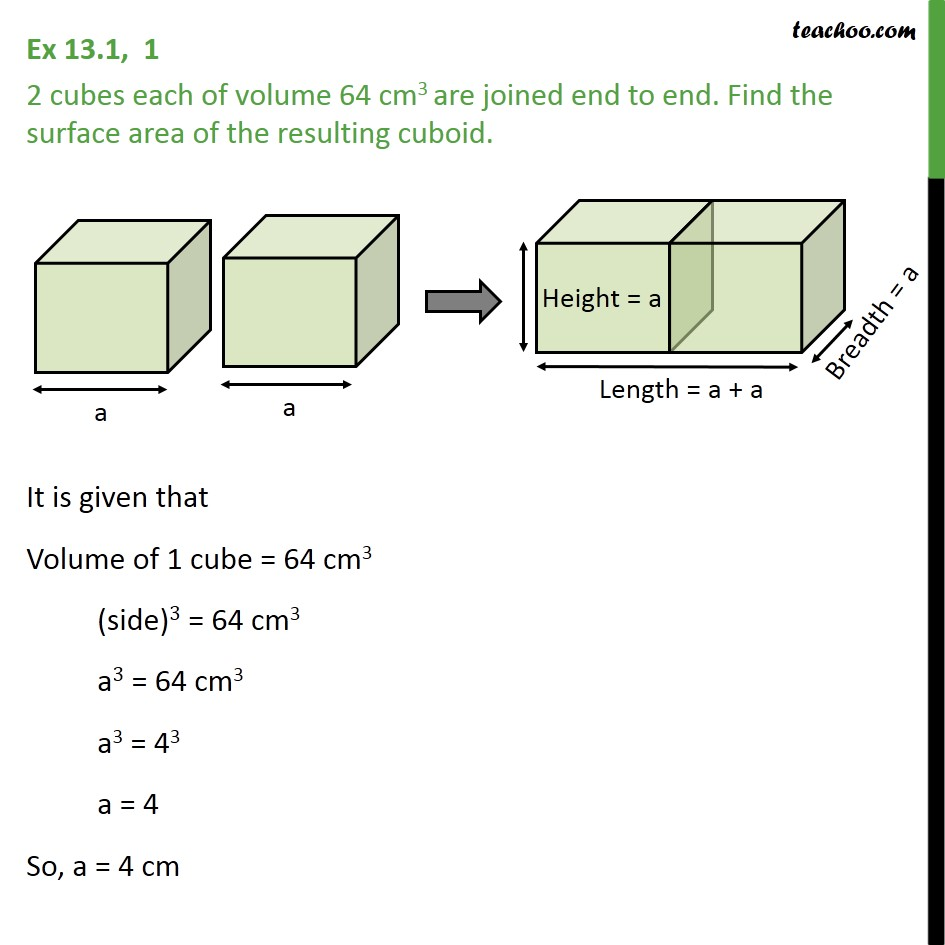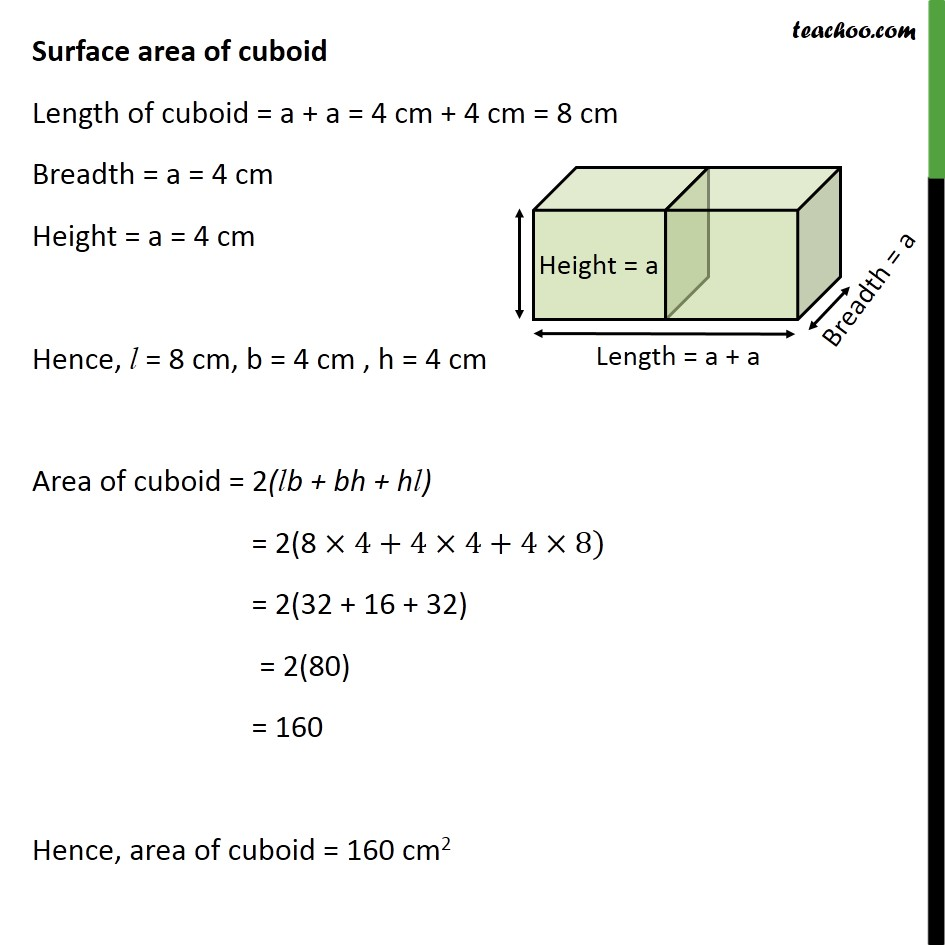1. Chapter 13 Class 10 Surface Areas and Volumes (Term 2)
2. Concept wise

Transcript

Ex 13.1, 1 2 cubes each of volume 64 cm3 are joined end to end. Find the surface area of the resulting cuboid. It is given that Volume of 1 cube = 64 cm3 (side)3 = 64 cm3 a3 = 64 cm3 a3 = 43 a = 4 So, a = 4 cm Surface area of cuboid Length of cuboid = a + a = 4 cm + 4 cm = 8 cm Breadth = a = 4 cm Height = a = 4 cm Hence, l = 8 cm, b = 4 cm , h = 4 cm Area of cuboid = 2(lb + bh + hl) = 2(8 4+4 4+4 8) = 2(32 + 16 + 32) = 2(80) = 160 Hence, area of cuboid = 160 cm2